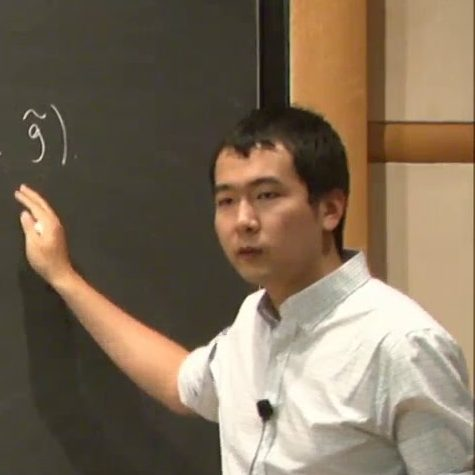Chao Li
(Courant Institute, NYU)
December 3, 2021
15:00
(EST)
Organized by Americas GNCG Seminar.

## Classifying sufficiently connected PSC manifolds in 4 and 5 dimensions

In this talk, I will discuss some recent developments on the topology of closed manifolds admitting Riemannian metrics of positive scalar curvature. In particular, we will prove if a closed PSC manifold of dimension 4 (resp. 5) has vanishing $\pi_2$ (resp. vanishing $\pi_2$ and $\pi_3$), then a finite cover of it is homotopy equivalent to $S^n$ or connected sums of $S^{n-1}\times S^1$. A key step in the proof is a homological filling estimate in sufficiently connected PSC manifolds. This is based on joint work with Otis Chodosh and Yevgeny Liokumovich.